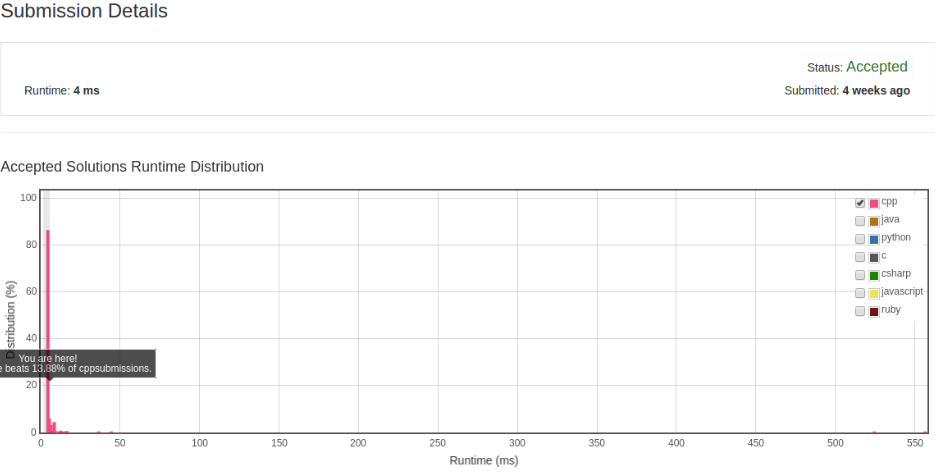# 凌云的博客

## LeetCode 算法题 27. Remove Element

### 题目

Given an array and a value, remove all instances of that value in place and return the new length.

Do not allocate extra space for another array, you must do this in place with constant memory.

The order of elements can be changed. It doesn't matter what you leave beyond the new length.

Example:

Given input array nums = [3,2,2,3], val = 3

Your function should return length = 2, with the first two elements of nums being 2.

``````Hint:

1. Try two pointers.
2. Did you use the property of "the order of elements can be changed"?
3. What happens when the elements to remove are rare?
``````

### 解法

``````class Solution {
public:
int removeElement(vector<int>& nums, int val) {
int i = 0, j = 0;
int size = nums.size();
for (; j != size; ++j) {
if (nums[j] != val) {
nums[i] = nums[j];
++i;
}
}

return i;
}
};
``````